# Artprice Methodology Explained

1- What is Artprice Indicator®?

The exclusive Artprice Indicator® gives you access, at the click of a mouse, to indicative values for an artwork in different years.

Artprice Indicator® generates a series of values, updated to the present, for a specified artwork. The indicative values are estimates of the potential value of a piece, based on the hammer price (excluding commission) at a specific date and the characteristics of the work. Indicative values are calculated from specific price indices using an algorithm unique in its field. Results can be displayed in euros or dollars.

Data are displayed as a table and plotted as historical charts, typically running from 1993 to 1 January 2004 and therefore including ten indicative values plus the hammer price. Note though, that a year may be dropped from the series where data does not allow a sufficiently precise indicative value to be calculated for that year. By default, Artprice Indicator® gives a minimum of four indicative values across ten years.

2- How Artprice Indicator® calculates indicative values

Indicative values are given as a monetary value, calculated by applying econometric equations to the artist's auction results held in Artprice's database.

Stage 1: calculating an index of prices specific to the work

For maximum precision, the methodology involves creating a range of price indices that can be applied to the work in question.
Indices are based on years with at least five relevant auction results. Only those results that share enough identifying characteristics in common with the work being valued are included in the calculation. If this threshold is not reached, no index is calculated for the year in question.

The price index is calculated using an econometric regression with specific modelling and estimates for each work. The econometric equation used is based on the technique known as hedonic price method.

By default the reference index is set for maximum precision, taking into account all the distinguishing characteristics of a work. Besides the signature, size and medium, the hedonistic pricing model also takes into account, where possible, the period the work dates from, the materials and technique used, and where the sale takes place.

Where information is missing (fewer than five auction results in the year sharing all the defining features of the work being valued), the precision of the calculation is reduced by one notch and the value included in the index for that year will then be taken from the price index for all works by the artist in that medium. If this still fails to produce enough results to construct a reliable index, the precision is lowered a further notch and the indicative value is based on the artist's work across all media, initially excluding prints, then, if necessary, with prints included.
The degree of accuracy of the calculation depends on the type of index on which the annual indicative value is based. This information is shown in an indicator called "degree of relevance".

The Artprice Indicator® does not take into account a number of points that may influence price movements, such as the state of preservation, origin, subject, framing, the circumstances applying at each auction etc. For a precise estimate we recommend that our clients take expert advice.

Stage 2: choice of index for each year

The precision of the calculation depends on the indices on which it is based.
The type of index used by the Artprice Indicator® is liable to change from one year to the next depending on the volume and quality of data available. Initially, Artprice Indicator® calculates the indicative value from the most precise price data available. If artwork price index (see definition below) is available for a particular year, Artprice Indicator® uses the next most precise index. The type of index selected can vary from year to year.

Artprice Indicator® works from three different index types:

• Artwork price index: an index of prices paid for works matching all the characteristics of the work (size, technique, materials, medium, date of creation). It is calculated solely from auctions of works by the same artist from the same period as the work being valued.
• Medium price index: index of prices paid for works matching the characteristics of the work to be valued (size, technique, materials, medium) based on auction prices in the same medium but including those from different periods.
• Artist price index: index of prices paid for works by the artist in all media. Prints are excluded by default unless the work being valued is itself a print.

If none of these indices can provide a value for a specific year or years, Artprice Indicator® returns no indicative value for the year or years in question.

Stage 3: calculating the degree of relevance

The quality of the indicative values may vary. The degree of index precision may vary not only from artist to artist and work to work, but also from year to year for the same work.

In the interest of transparency Artprice Indicator® provides a benchmark indicating the precision of the values calculated: the degree of relevance.

The Artprice Indicator® degree of relevance takes into account:

• The overall precision of the estimate provided by the equation
• The number of auction results on which the calculation was based. The larger the number of results, the lower the risk of a biased estimate
• The type of index used in the calculation
• The significance (in econometric terms) of the time variable in the price equation
• The volatility of the price index over time as a function of the size of temporal fluctuations.

The degree of relevance is calculated individually for each year studied.

For comparison purposes, the degree of relevance is derived by merging several variables, all expressed as values ranging from 0 to 1.

Quantitatively, the variables used are as follows:

• c1 = the price equation's coefficient of determination R² (ranges from 0 to 1). The coefficient of determination (also called the multiple correlation coefficient) explains how good a fit the model is. It measures how much of the variation in price is explained by the price regression. The closer it is to 1, the better the model's fit: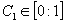• c2 = variable reflecting the estimate's degree of freedom (dof). This concept reflects the number of usable values left after carrying out a statistical estimate. For instance, if we have 120 usable auction results and the estimate involves 10 parameters the degree of freedom is 110. Here the values used are: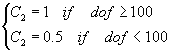• c3 = variable reflecting the type of index used in the calculation.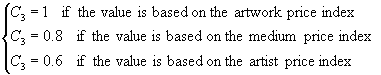• c4 = variable reflecting the significance of the estimated parameter affecting the temporal variable for the year in question in the hedonistic pricing equation. This variable is derived from the probability of rejection when the parameter is tested for nullity (student test):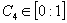• c5 = variable reflecting the volatility of the price index. We assume that the greater the fluctuation in the price index, the greater the risk of error. To estimate price volatility we calculate a first order auto-correlation coefficient, c5: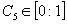Finally, the degree of relevance is calculated using the variable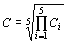In the interest of simplicity the result is expressed as a star rating.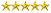=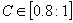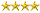=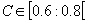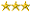=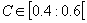More stars mean a more precise Artprice Indicator®
Any values where the degree of relevance falls below our qualitative threshold of 0.4 are excluded from the calculation.

Clearly errors are still possible even with the level of precision applied, as an auction result will also depend on the circumstances of the sale and factors specific to the work in question (state of preservation, origin, etc.) which are not included in our calculations. What we provide here are "average" annual values. To obtain a precise estimate of an artwork's value, Artprice recommends that its clients take expert advice.

## Compare products

{"one"=>"Select 2 or 3 items to compare", "other"=>"{{ count }} of 3 items selected"}

Select first item to compare

Select second item to compare

Select third item to compare